Author: Oscar Cronquist Article last updated on April 24, 2019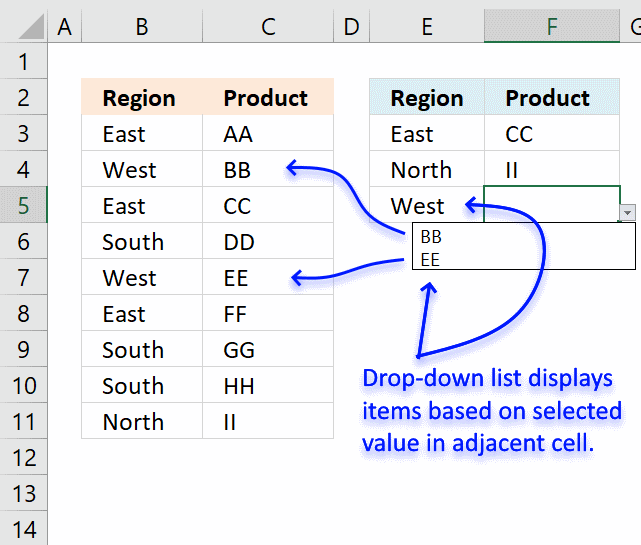This article demonstrates how to set up dependent drop-down lists in multiple cells. The drop-down lists are populated based on the adjacent value on the same row, in other words, they are dependent on each other. Select Region "East" demonstrated above and you can only select Products "BB" and "EE", the table in cell range B2:C11 determines how they are related.

Regular drop-down lists used here are easy to insert and customize, the formulas in this workbook are easy to create and so are the named ranges as well. I will in detail explain how they work.

The downside with this approach is that you can only use two connected drop-down lists. For example, three dependent drop-down lists actually require a three-dimensional table which is possible using VBA, however, outside the scope of this article. Note, to be clear, there is no VBA code in the example demonstrated here.

Another downside with regular drop-down lists is that if you select a Region value and then a Product value and then go back and change the Region value to another value the Product value stays the same. It would have been better if that value became blank in those occasions.

It is also possible to paste a value and overwrite the drop-down list, this is how they are designed and nothing I can do about more than using perhaps VBA code or Event code to prevent such scenarios.

Check out the Dependent Drop Down lists Add-In if you need more than two dependent drop-down lists.

### How I built this workbook

Cell range B2:C11 contains an Excel defined Table that automatically expands or shrinks when values are added or deleted respectively, you don't need to adjust cell references each time you edit the Excel defined Table which is a huge time saver.

There is also another data set, shown below, that is built using two array formulas. The data set extracts items (Products) for each possible value (Region) which makes it easier to populate the drop-down lists using only formulas and named ranges.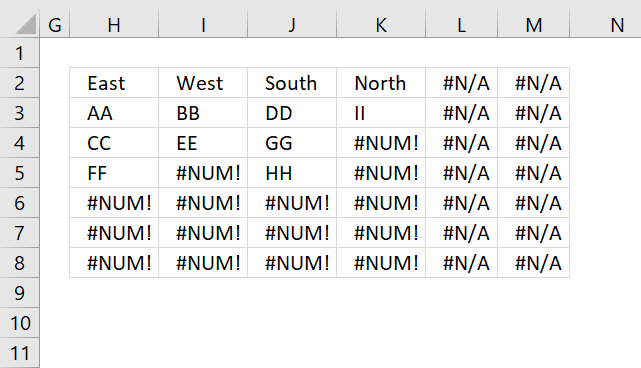This table is dynamic and changes instantly whenever the source table is edited. Don't worry, I will explain the table above and the formulas used later in this article.

#### Excel defined Table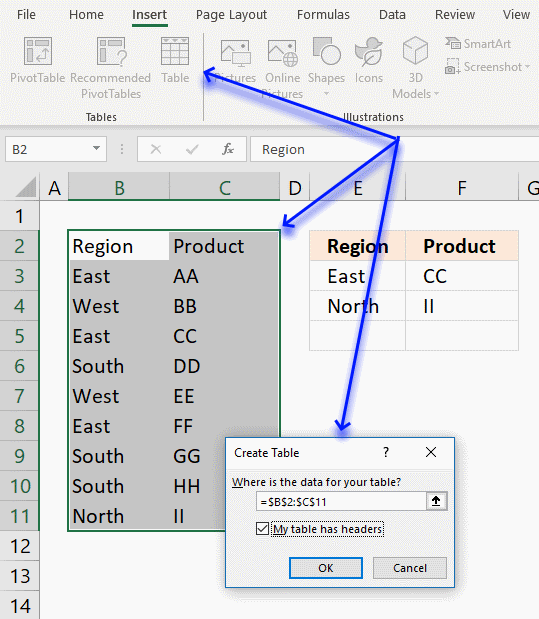Here is how to create an Excel defined Table:

1. Select cell range B2:C11.
2. Go to tab "Insert" on the ribbon.
3. Click "Table" button.
5. Click OK button.

The main reason I am using an Excel defined Table here is that you can use a "structured reference" which is basically a table name instead of a regular cell reference.

#### Relationships between categoriesThe first array formula returns unique distinct values in cell H2:M2 from named range Region. The second array formula in cell range H3:M7 returns coherent values from each region. All of these calculations can be hidden or placed on a calculation sheet.

Array formula in cell H2:

=INDEX(Table1[Region], MATCH(0, COUNTIF(\$G\$2:G2, Table1[Region]), 0))

To enter an array formula, type the formula in a cell then press and hold CTRL + SHIFT simultaneously, now press Enter once. Release all keys.

The formula bar now shows the formula with a beginning and ending curly bracket telling you that you entered the formula successfully. Don't enter the curly brackets yourself.

Note, the formula above contains a cell reference that changes when you copy the cell and paste it to cell range H2:M2.

Array formula in cell H3:

=INDEX(Table1[Product], SMALL(IF(H\$2=Table1[Region], MATCH(ROW(Table1[Region]), ROW(Table1[Region]))), ROWS(\$A\$1:A1)))

Copy cell H3 and paste to cell range H3:M8, if you have more categories (Regions) and Items (Products) than this example you may have to extend the formulas to a larger cell range accordingly.

#### Named ranges

Now it is time to create the two last named ranges that will be used in data validation lists.

1. Select cell F3, this step is very important because the named ranges contain formulas that contain relative cell ranges.
2. Go to tab "Formula" on the ribbon.
3. Click the "Name Manager" button and a dialog box appears.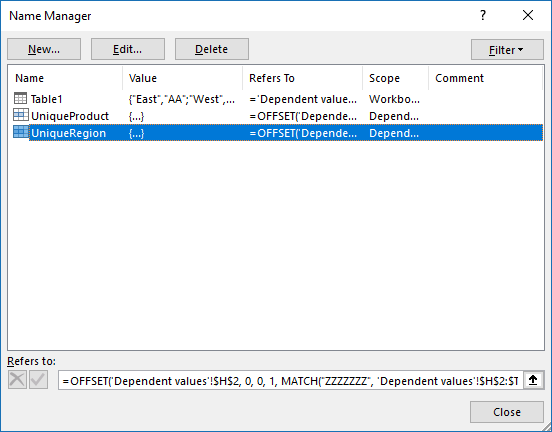4. Click "New..." button.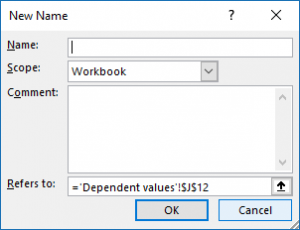5. Enter the name UniqueRegion shown below.
6. Enter the formula.
7. Click OK button.
8. Repeat step 3 to 6 with UniqueProduct

Name: UniqueRegion

Formula:

=OFFSET('Dependent values'!\$H\$2, 0, 0, 1, MATCH("ZZZZZZZ", 'Dependent values'!\$H\$2:\$T\$2))

Name: UniqueProduct

Formula:

=OFFSET('Dependent values'!\$H\$3, 0, MATCH('Dependent values'!\$E3, 'Dependent values'!\$H\$2:\$T\$2, 0)-1, SUMPRODUCT(--('Dependent values'!\$E3=Table1[Region])))

You can find an explanation to the dynamic named ranges displayed above in the article below.

Create a dynamic named range

A dynamic named range grows automatically when new values are added and also shrinks if values are deleted. This saves […]

#### Data validation lists

The following steps shows you how to create regular drop-down lists in column E, linked to a dynamic named range UniqueRegion.

1. Select cell E3
2. Go to tab "Data"
3. Click "Data validation" button
4. Select "List"
5. Type =UniqueRegion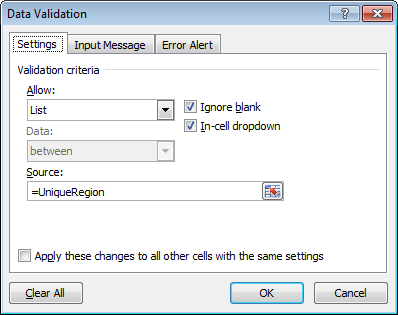6. Click OK
7. Copy cell E3 and paste to cells below as far as needed.

The following steps shows you how to create regular drop-down lists in column F, linked to a dynamic named range UniqueProduct.

1. Select cell F3
2. Go to tab "Data"
3. Click "Data validation" button
4. Select "List"
5. Type =UniqueProduct
6. Click OK
7. Copy cell F3 and paste to cells below as far as needed.